# The product geometry $S^2 \times \mathbb E$

## What is $\mathbb S^2\times\mathbb{E}$

This geometry is the cartesian product of the two-sphere and the real line. It can be also seen as the universal cover of the product $M = S^2 \times S^1$ where $S^2$ is the two-sphere and $S^1$ the unit circle.

Click on the button below to reveal a concrete model of $S^2 \times \mathbb E$.

The two-sphere is the unit sphere in the 3-dimensional euclidean space. It can be described as $$Y = \left\{ (x,y,z) \in \mathbb R^3 \mid x^2 + y^2 + z^2 = 1 \right\}$$ endowed with the following rieamanian metric $$ds^2 = dx^2 + dy^2 + dz^2.$$

Consequently a possible model of $S^2 \times \mathbb E$ is the following subset $X$ of $\mathbb R^4$ $$X = \left\{ (x,y,z,w) \in \mathbb R^3 \mid x^2 + y^2 + z^2 = 1 \right\}$$ endowed with the following rieamanian metric $$ds^2 = dx^2 + dy^2 + dz^2 + dw^2.$$ The isometry group of $X$ is $O(3) \times {\rm Isom}(\mathbb R)$ where $O(3)$ is the isometry group of $S^2$ and ${\rm Isom}(\mathbb R) = \mathbb R \rtimes \mathbb Z/ 2 \mathbb Z$ is the isometry group of the real line.

## Some views of $\mathbb S^2\times\mathbb{E}$

Warning: Some of the real-time simulations below requires a powerful graphic card. If your computer is not fast enough, you can reduce the size of your browser window. Click on the button below to reveal the fly commands.

The default controls to fly in the scene are the following. You can choose your keyboard in the Option controls pannel in the top right corner of the window

Command QWERTY keyboard AZERTY keyboard
Yaw left a q
Yaw right d d
Pitch up w z
Pitch down s s
Roll left q a
Roll right e e
Move forward arrow up arrow up
Move backward arrow down arrow down
Move to the left arrow left arrow left
Move the the right arrow right arrow right
Move upwards ' ù
Move downwards / =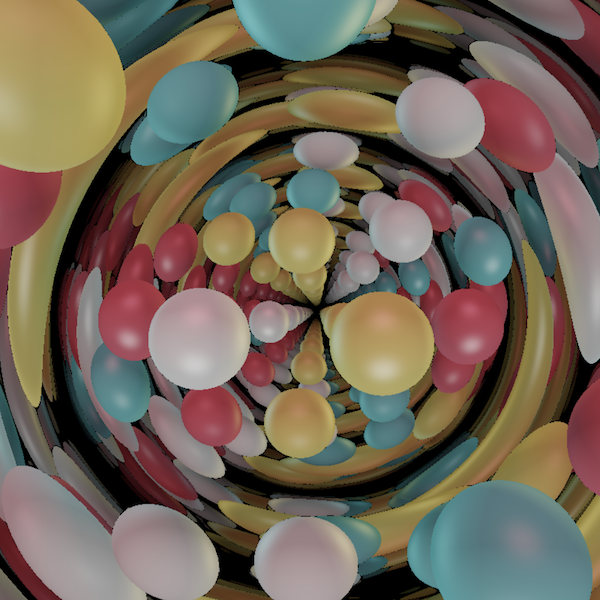##### Spheres

Twelve Spheres in S2xS1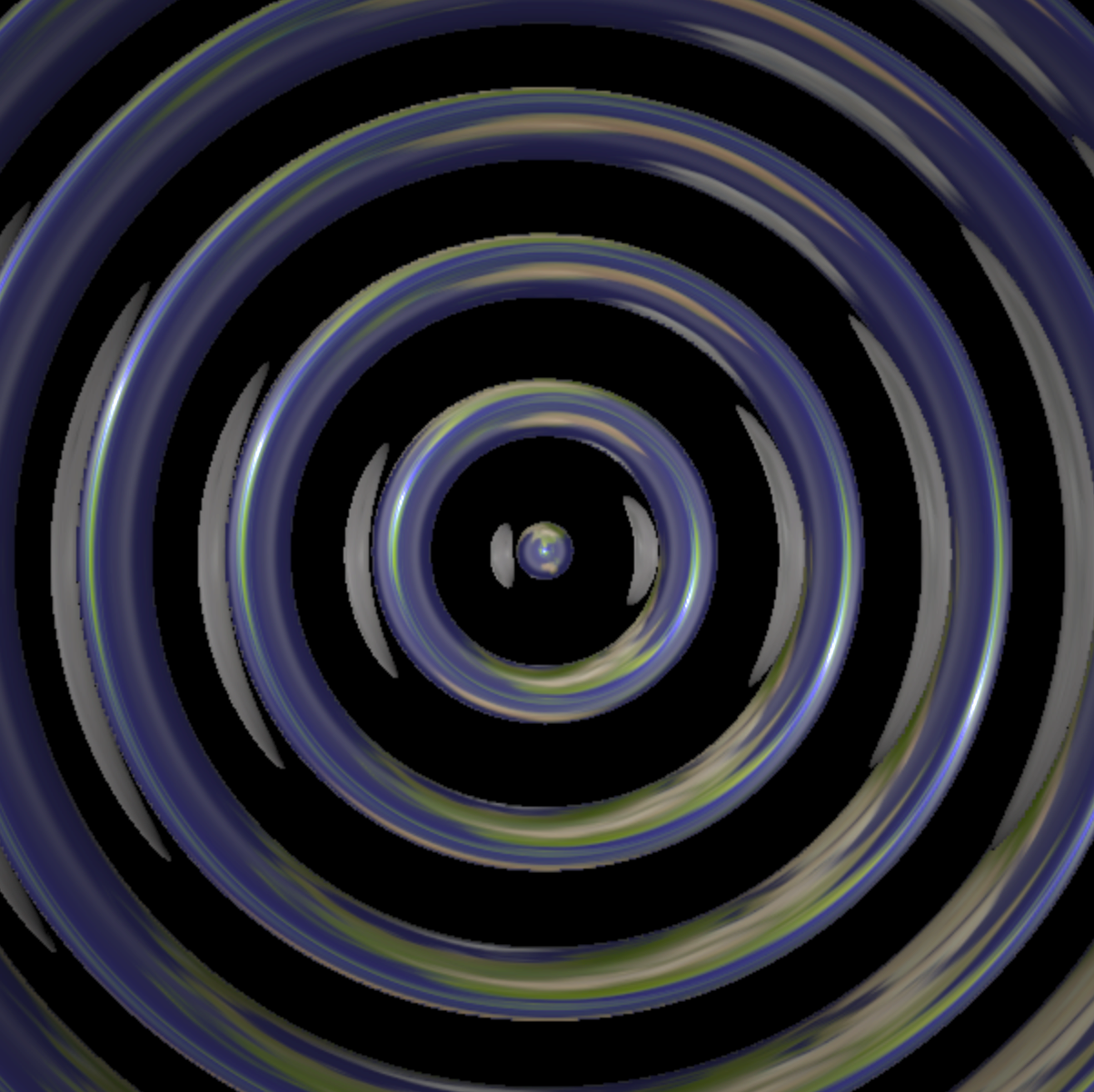##### Seeing in S2xE

The earth and moon, to provide a familiar context in a strange space.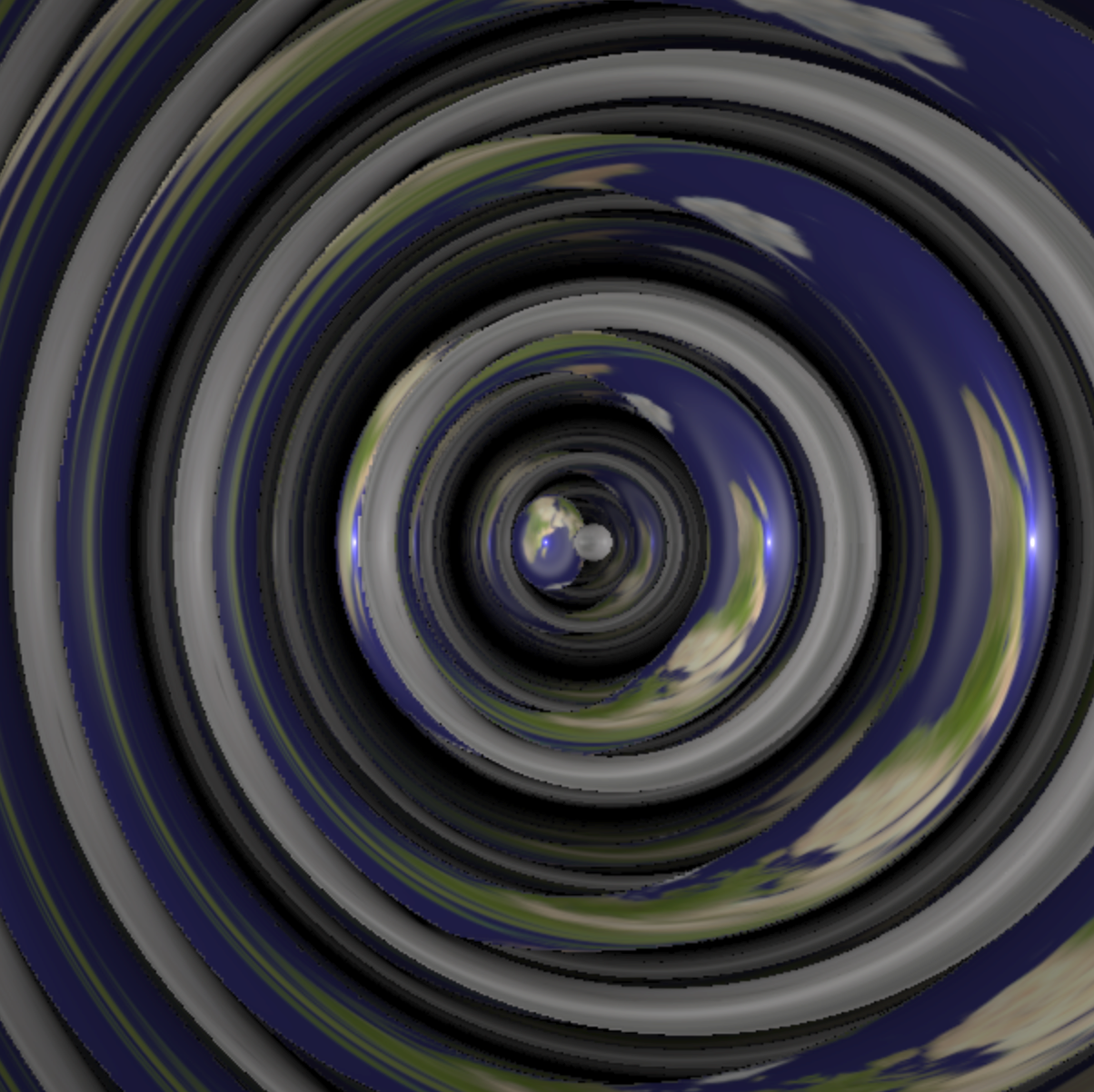##### Seeing in S2xS1

The earth and moon, to provide a familiar context in a strange space.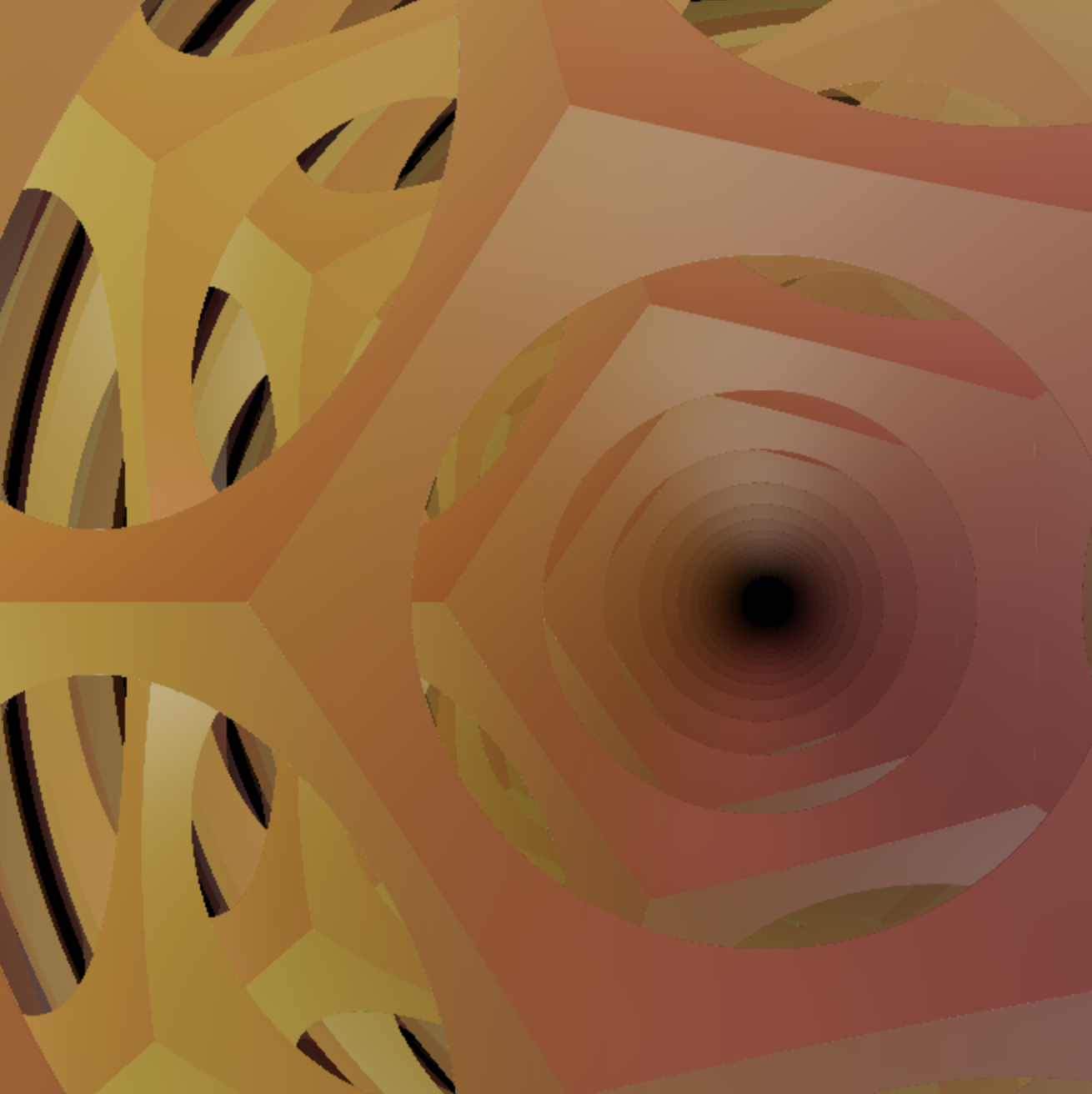##### A Dodecahedron in S2xS1

Totally Geodesic Spheres

HD pictures of $\mathbb S^2 \times \mathbb E$ can be found in the gallery

## Features of $\mathbb S^2\times\mathbb{E}$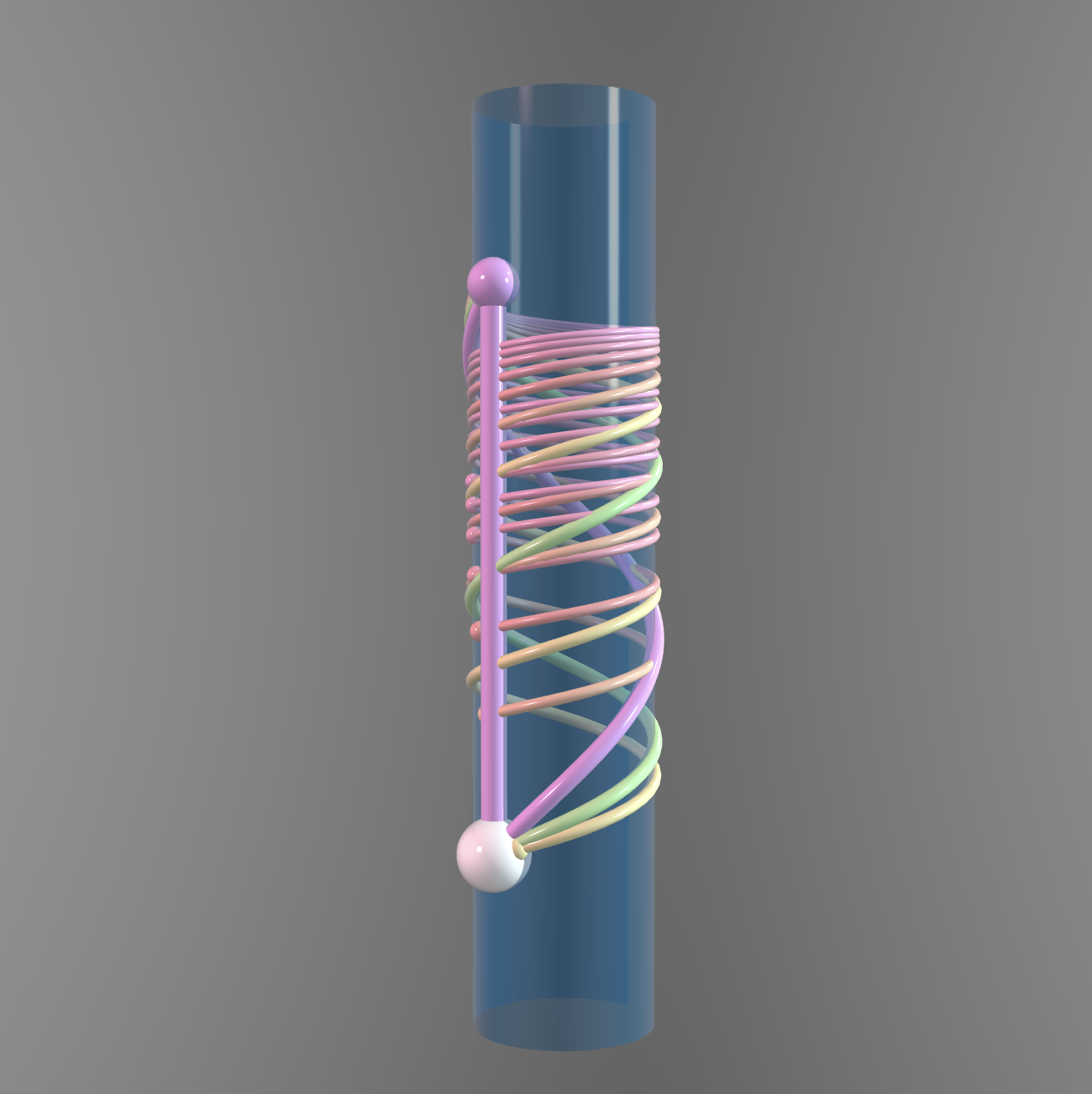##### Understanding the Exponential Map

Geodesics on a Cylinder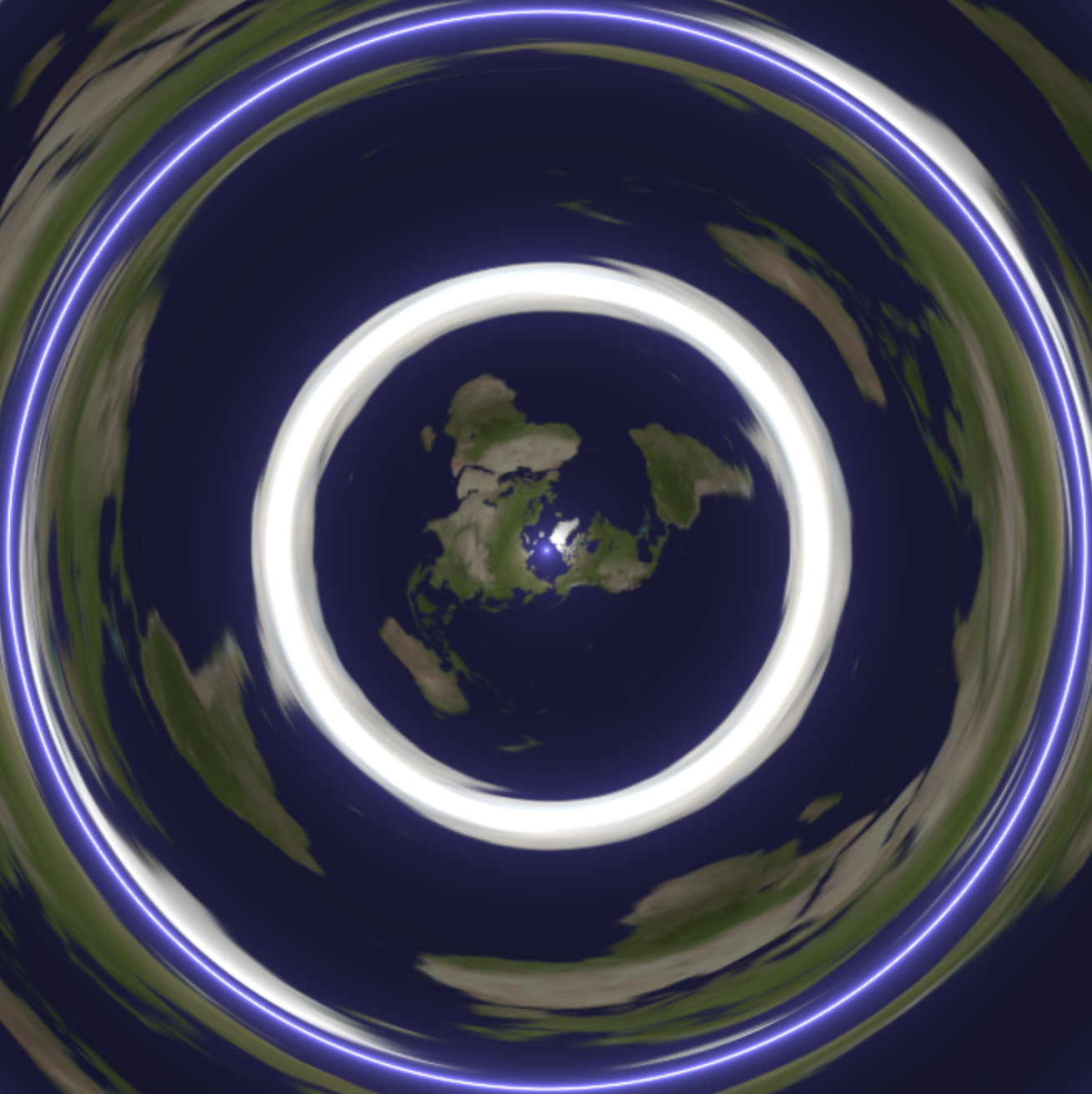##### Equatorial Spheres in $\mathbb{S}^2\times\mathbb{E}$

Totally Geodesic Spheres